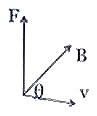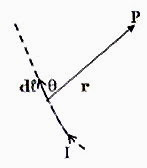## Pages

`“Life is like riding a bicycle.  To keep your balance you must keep moving.”–Albert Einstein`

## Friday, February 1, 2008

### AP Physics B & C - Magnetic Field - Equations to be Remembered

The section ‘Magnetic Fields’ in the AP Physics syllabus contains the following sub sections:

(1) Forces on moving charges in magnetic fields

(2) Forces on current-carrying wires in magnetic fields

(3) Fields of long current-carrying wires

(4) Biot-Savart’s law and Ampere's law (For AP Physics C only)

AP Physics B carries 4% of the total points in this section while AP Physics C carries 10%.

Here are the equations to be remembered in this section:

(1) The magnetic force ‘F’ acting on a particle of charge ‘q’ moving with velocity ‘v’ making an angle ‘θ’ with a magnetic field ‘B’ (fig.) is given by

F = qvB sinθ

The force F is perpendicular to both v and B. In vector form, the above equation is

F = qv×B

Note that bold face characters are used to represent vectors.

When electric and magnetic fields act simultaneously on a charge, the total force on the charge is given by Lorentz force equation,

F = q(v×B + E) where E is the electric field.

(2) The path of a charged particle of mass ‘m’ projected with a velocity ‘v’ perpendicular to a magnetic field B is a circle of radius ‘r’ given by

qvB = mv2/r, where ‘q’ is the charge

[Note that we have equated the magnetic force to the centripetal force]

Therefore, r = mv/qB

If the particle is projected into the magnetic field at an angle other than zero or 90º, the path is a helix of radius ‘r’ given by

r = mv sinθ/qB

(3) The period of circular motion as well as helical motion of a charged particle in a magnetic field is

T = 2πm/qB

(4) The frequency of revolution along the circular path (or helical path) is

f = qB/2πm

This is called the cyclotron frequency

(5) The magnetic force ‘dF’ acting on an elemental length dℓ of a conductor carrying a current ‘I’ placed in a magnetic field B, making an angle ‘θ’ with the magnetic field is given

dF = IdℓB sinθ

The force dF is perpendicular to both dand B. In vector form, the above equation is

dF = I d×B, treating the elemental length dℓ as a vector.

In the case of a straight conductor of length ℓ, the magnetic force is

F= IℓB sinθ which can be written in vector form as

F = I ×B

(6) Force per unit length between two infinitely long parallel current carrying conductors is given by

F = µ0I1I2/2πd, where µ0 is the permeability of free space, ‘d’ is the separation between the conductors and I1 and I2 are the currents in the conductors.

(7) Torque on a plane current carrying coil (current loop) placed in a magnetic field B is

τ = nIAB sinθ, where ‘n’ is the number of turns in the coil, A is the area of the coil, I is the current in the coil and θ is the angle between the area vector and the magnetic field vector. Remember that the area is a vector which has magnitude equal to the area and direction perpendicular to the area.

(8) Magnetic field (dB) due to a current element of length dℓ at a point P distant ‘r’ fromthe current element is given by Biot-Savart law:
dB = (µ0/4π) Id sinθ/r2

This magnetic field is perpendicular to the plane containing the current element and the point P.

In vector notation the above equation is

dB = (µ0/4π) Id ×r/r3

Here r is a vector of length r directed from the current element to the

The length of the current element also is treated as a vector dof length dℓ with its direction same as that of the current.

(9) The magnetic field due to a straight infinitely long current carrying conductor
at a point P at a perpendicular distance ‘r’ from the conductor is
B =µ0I/2πr

(10) The magnetic field due to a plane circular current carrying

coil of ‘n’ turns and radius R at a point P on the axis at a distance

‘x’ from the centre of the coil is

B = µ0nR2I /2(R2 + x2)3/2

The magnetic field at the centre of the coil is

B= µ0nI/2R

(11) The magnetic field on the axis of an infinitely long straight solenoid at a point P well within the solenoid is

B= µ0nI, where ‘n’ is the number of turns per metre of the solenoid.

(12) The magnetic field inside a toroid of ‘n’ turns per metre is

B= µ0nI

(13) Ampere’s circuital law states that the line integral of magnetic flux density over any closed curve is equal to µ0 times the total current passing through the surface enclosed by the closed curve. This is stated mathematically as

B. d = µ0I (The integration is over the closed path)

Amperes circuital law as modified by Maxwell to accommodate the displacement current flowing through even free space is

B. d = µ0 [I+ ε0 (dφE/dt)], where ε0 (dφE/dt) is the displacement current resulting from the rate of change of electric flux φE. ε0 is the permittivity of free space.

In the next post we will discuss questions in this section.